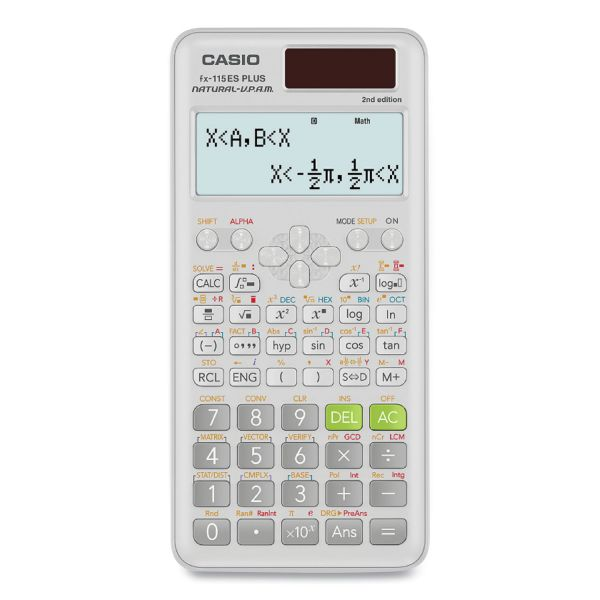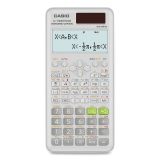# Casio FX-115ESPLS2-S 2nd Edition Scientific Calculator, 12-Digit Natural Textbook Display

Product Number: CSOFX115ESPLS2
• Natural Textbook Display shows formula and results exactly as they appear in the textbook and displays formulas and results simultaneously for 460 built-in functions.
• Can transform a displayed decimal calculation result to a fraction or mixed number. It can also be converted back to the decimal form.
• Perform addition, subtraction, multiplication and division.
• Augment and absolute value calculations. Reciprocal, square and cube calculations. Conjugate complex number calculations.
More details
\$26.50
Free Shipping Orders Over \$45+ Estimated Delivery  Fri 10/22  Mon 10/25   (3 business days)
Favorite
Compare
Request Quote

## Product Description

Casio FX-115ESPLS2-S 2nd Edition Scientific Calculator, 12-Digit Natural Textbook Display - Casio FX-115ESPLS2-S 2nd Edition Scientific Calculator with slide on hard case and sleek new design. With the Natural Textbook Display, in addition to advance Statistics, Matrix, and Conversion functionality, is a perfect choice for high school and college students. Recommended for students taking General Math, Pre-Algebra, Algebra I and II, Geometry, Trigonometry, Statistics, Calculus, Chemistry, Engineering.

• Natural Textbook Display shows formula and results exactly as they appear in the textbook and displays formulas and results simultaneously for 460 built-in functions.
• Can transform a displayed decimal calculation result to a fraction or mixed number. It can also be converted back to the decimal form.
• Perform addition, subtraction, multiplication and division.
• Augment and absolute value calculations. Reciprocal, square and cube calculations. Conjugate complex number calculations.
• Performs linear regression, quadratic regression, logarithmic regression, e exponential regression, ab exponential regression, power regression and inverse regression.
• Solve simultaneous linear equations with two unknowns, simultaneous linear equations with three unknowns, quadratic equations and cubic equations.
• The Matrix mode allows you to perform matrix operations on matrices with up to 3 rows and 3 columns.Guides & Information
Related Articles

### Product Specifications

• +/- Switch Key
No
• Amortization
No
• Assembly Required
No
• Backspace Key
Yes
• Base Number Calculations
No
• Bond Calculations
No
• Brand Name
Casio
• Case
Slide-On Hard-Case
• Cash Flow Calculations
No
• Complex Number Calculations
No
• Confidence Interval Calculating
No
• Cost/Sell/Margin
No
• Country of Origin
TH
• Currency Exchange Function
No
• Date Calculations
No
• Decimal Function
Yes
• Depreciation Calculations
No
• Display Angle
Fixed
• Display Characters Height
4 mm
• Display Characters x Display Lines
12 x 2
• Display Notation
Natural Textbook Standard Scientific
• Display Type(s)
LCD
• Double Zero Key
No
• Entry Logic
V.P.A.M.
• Equation Editor
Yes
• Fraction/Decimal Conversions
Yes
• Fraction Calculations
Yes
• Global Product Type
Calculators-Scientific
• Grand Total Key
No
• Graphing Functions
No
• Higher Mathematical Functions
Advanced Statistical Distribution Calculations Base-N Derivatives Inequalities Integrals Matrices Vectors Verify
• Hyperbolic Functions
Yes
• Hypothesis Testing
Yes
• I/O Port
No
• Interest Rate Conversion
No
• Item Count Function
No
• Linear Regression
Yes
• Loan Calculation
No
• Logical (Boolean) Operations
No
• Markup/Down Key
No
• Matrices
No
• Memory
1 Independent 8 Constant
• Metric Conversion
No
• Number of Display Digits
12
No
• Percent Key(s)
No
• Polar-Rectangular Conversion
No
• Post-Consumer Recycled Content Percent
0%
• Power Source(s)
Battery Solar
• Pre-Consumer Recycled Content Percent
0%
• Probability (Random Number)
Yes
• Recycled
No
• Replacement Batteries
LR44
• Simultaneous Equations
No
• Size
3.15 x 6.37
• Square Root Key
Yes
• Tax Calculation
No
• Time-Value-of-Money
No
• Time/Date
No
• Total Recycled Content Percent
0%
• Trig/Log Functions
Yes
• Variable Regression
Yes
• Variable Statistics
Yes
• Wall-Mountable
No